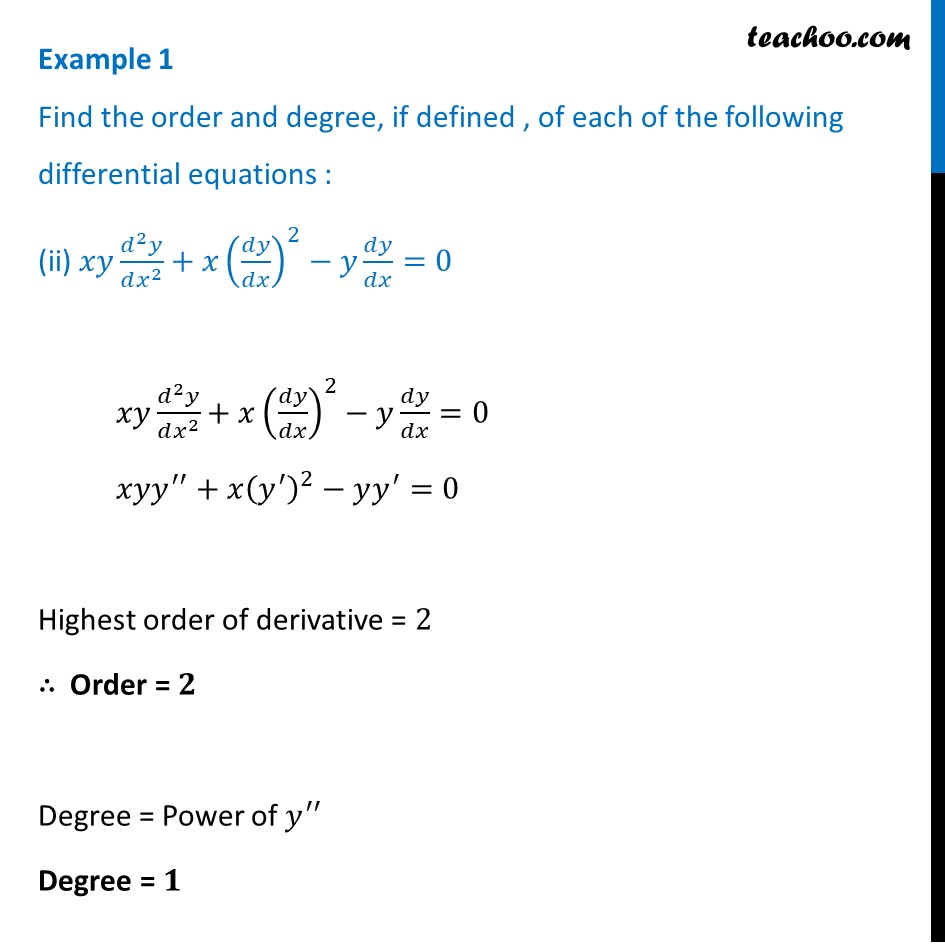Examples

Chapter 9 Class 12 Differential Equations
Serial order wiseLearn in your speed, with individual attention - Teachoo Maths 1-on-1 Class

### Transcript

Example 1 Find the order and degree, if defined , of each of the following differential equations : (ii) 𝑥𝑦 (𝑑^2 𝑦)/(𝑑𝑥^2 )+𝑥(𝑑𝑦/𝑑𝑥)^2−𝑦 𝑑𝑦/𝑑𝑥=0 𝑥𝑦 (𝑑^2 𝑦)/(𝑑𝑥^2 )+𝑥(𝑑𝑦/𝑑𝑥)^2−𝑦 𝑑𝑦/𝑑𝑥=0 𝑥𝑦𝑦^′′+𝑥(𝑦^′ )^2−𝑦𝑦^′=0 Highest order of derivative = 2 ∴ Order = 𝟐 Degree = Power of 𝑦^′′ Degree = 𝟏